# Quiz 11: Probability and Statistics

Business

Objective Probability - The probability of a specific outcome (in a given a set of outcomes for an activity) is the ratio of the number of specific outcomes to the total number of outcomes. This probability can be determined before the event occurs. Subjective Probability - This probability is a prediction that carries the predictor's judgment with it. a. The forecast of snow is based on the meteorologist's experience and expert analysis of the weather conditions. Therefore, the meteorologist's prediction is based on his judgment. This is a subjective probability. b. The probability of catching fish is based on the fisherman's experience and expert analysis of fishing conditions (weather, water depth, bait available, etc). Therefore, the fisherman's prediction is based on his judgment. This is a subjective probability. c. The probability of the prime interest rate rising in the coming year is based on the data of previous years and the manager's expert analysis of the data based on his experience. Therefore, this is both a subjective and objective probability. d. The probability of a certain team winning the World Series is based on the sportswriter's knowledge of the teams involved in the World Series that year. The prediction is based on the judgment of the sportswriter. This is a subjective probability. e. The probability of demand for a product will be a specific amount next month is based on data from previous months of the demand on that product. This probability can be determined before the event occurs, and is an objective probability. f. The probability of a certain candidate winning the election is based on the predictor's knowledge of the candidates involved in the election. The prediction is based on the judgment of the predictor. This is a subjective probability. g. The probability of a machine breaking down is based on data from the history of the machine breaking down. This probability can be determined before the event occurs, and is an objective probability. h. The probability of being dealt four aces is the ratio of the number of aces to the total number of cards in the deck. This probability can be determined before the event occurs, and is an objective probability.

Lower limit= 314.1304-1.645 * 102.588/v92 Lower limit= 296.5 Similarly, calculate the upper limit as shown below: Upper limit=314.1304-1.645 * 102.588/v92 Upper limit= 331.7 Thus, the average daily attendants of 320 lie between the interval (296.5, 331.7) and the criteria is met. 3. Calculate the mean and standard deviation for the weekend values, as shown below: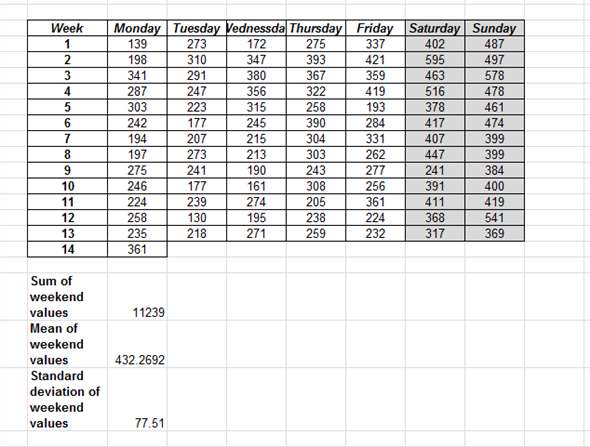Apply the probability for the current average weekend attendance to be not more than 500, as shown below: At the 90% confidence interval the upper and the lower limits are: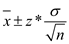Here, Z=1.645 at 90%confidence interval s= standard deviation n= number of observations Apply the formula to obtain the limits as below: Lower limit= 432.26-1.645 * 77.51/v26 Lower limit= 406.3 Similarly, calculate the upper limit as shown below: Upper limit=432.26-1.645 * 77.51/v26 Upper limit= 458.3 Thus, the average weekend an attendant of 500 does not lie between the interval (406.3, 458.3) and the criteria is not met. Thus, the club should not undertake the issue of new shares as the criteria is not met.

a. The probability of being dealt a face card is the ratio of the number of face cards to the total number of cards in the deck. There are 12 face cards in a deck of 52 cards. The probability is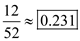. b. The probability of being dealt a queen is the ratio of the number of queens to the total number of cards in the deck. There are 4 queens in a deck of 52 cards. The probability is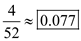. c. The probability of being dealt a spade is the ratio of the number of spades to the total number of cards in the deck. There are 13 spades in a deck of 52 cards. The probability is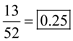. d. The probability of being dealt a jack of spades is the ratio of the number of jack of spades to the total number of cards in the deck. There is one jack of spades in a deck of 52 cards. The probability is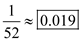.

There is no answer for this question